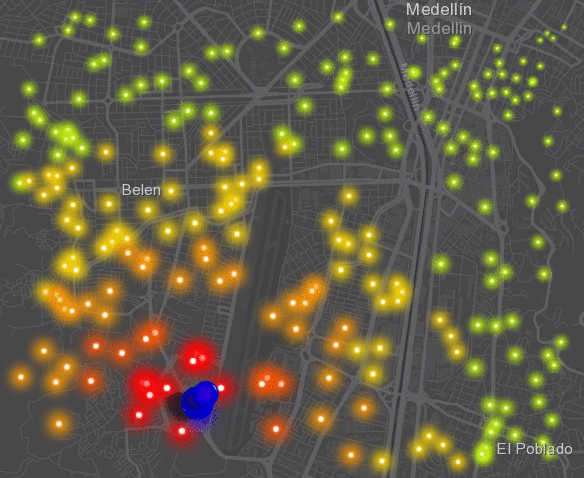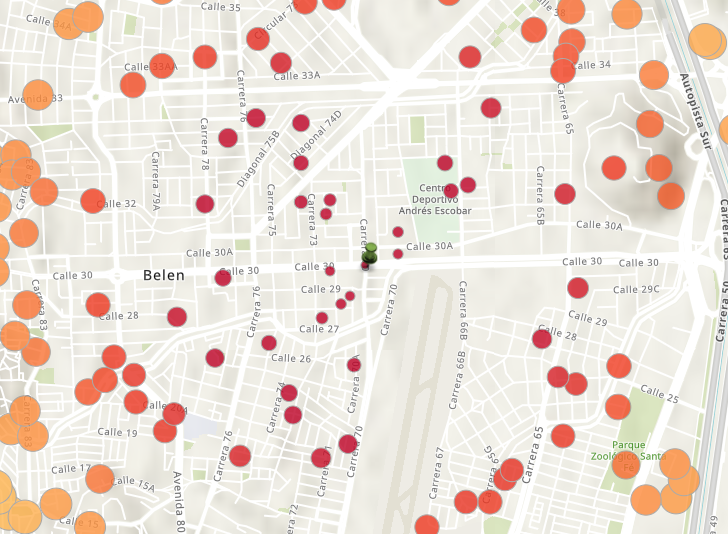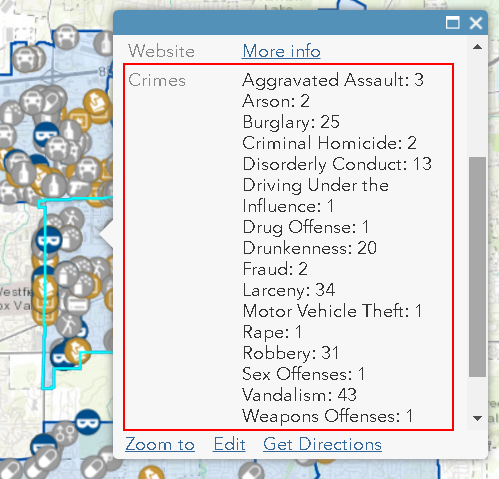# Using Arcade Geometry functions in ArcGIS Online

6721
19
12-12-2017 11:49 AMbyEsri Esteemed Contributor

I noticed that the December update of ArcGIS Online included Geometry Functions | ArcGIS for Developers . That is really swell, but I am trying to get my head around this and without the actual access to other features, I have problems seeing the benefits to this.

Kelly Gerrow‌; or anyone else, is it possible provide some real-world examples of how this can be used. I suppose I could hard code another geometry storing the json in the arcade expression, but it does not seem as the right way to do this.

Especially the SetGeometry intrigues me  ( Geometry Functions | ArcGIS for Developers ) I can imagine this it returns an error if I buffer a point feature and try to set the geometry, but when I apply it on a polygon, it returns that the geometry is immutable.

Any example would be highly appreciated!

1 Solution

Accepted SolutionsbyEsri Esteemed Contributor

To make things a bit more dynamic (still on a hard coded geometry) I created an expression of a buffer of a point that grows in time and each point is given a symbology based on the distance from that growing circle:

``````var start_time = Date(2017, 11, 14, 10, 10, 0);
var curr_time = Now();
var dif_sec = DateDiff(curr_time, start_time, "seconds");
var buf_dist = Abs(dif_sec);

var json_pnt = '{"x":-8415299.294,"y":692928.204,"spatialReference":{"wkid":102100,"latestWkid":3857}}';
var source_pnt = Point(json_pnt);
var pnt = Geometry(\$feature);
var buf = Buffer(source_pnt, buf_dist, "meter");

var dist = Distance(pnt, buf, 'meters');

var result = "";
if (dist == 0) {
result = "Danger!";
} else if (dist <= 500) {
result = "Warning: 0 - 500m";
} else if (dist <= 1000) {
result = "Warning: 500m - 1km";
} else if (dist <= 1500) {
result = "Warning: 1km - 1.5km";
} else if (dist <= 2500) {
result = "Warning: 1.5km - 2.5km";
} else {
result = "No danger (>2.5km)";
}

return result;
‍‍‍‍‍‍‍‍‍‍‍‍‍‍‍‍‍‍‍‍‍‍‍‍‍‍‍‍‍‍‍‍‍‍‍‍‍‍‍‍‍‍‍‍‍‍‍‍‍‍‍‍‍‍‍‍‍``````

Another one for the size of the symbol (inverted in this case to have larger symbols for those point closer to the circle):

``````var start_time = Date(2017, 11, 14, 10, 10, 0);
var curr_time = Now();
var dif_sec = DateDiff(curr_time, start_time, "seconds");
var buf_dist = Abs(dif_sec);

var json_pnt = '{"x":-8415299.294,"y":692928.204,"spatialReference":{"wkid":102100,"latestWkid":3857}}';
var source_pnt = Point(json_pnt);
var pnt = Geometry(\$feature);
var buf = Buffer(source_pnt, buf_dist, "meter");

var dist = Distance(pnt, buf, 'meters');

return dist;
‍‍‍‍‍‍‍‍‍‍‍‍‍‍‍‍‍‍‍‍‍‍‍‍‍‍‍``````

Which results in something like this when you make use of the new Firefly symbols available in ArcGIS Online:19 RepliesbyEsri Esteemed Contributor

Simple example of what can be done could be visualizing the distance to a given point, using this simple script:

``````var json_pnt = '{"x":-8414809.305,"y":695061.198,"spatialReference":{"wkid":102100,"latestWkid":3857}}';
var given_pnt = Point(json_pnt);

var pnt = Geometry(\$feature);
var dist = Distance(pnt, given_pnt, 'meters');

return dist;‍‍‍‍‍‍‍``````

With this result (if I use the distance for size and color):This might be interesting if the points are dynamic and provided by for instance GeoEvent in real time, but the fact that I have to include a hard coded point in the expression does not seem right to me.Occasional Contributor

I would like to symbolise a point hosted feature layer based on whether they intersect a polygon hosted feature layer in the same map using the Intersects function

### Intersects

##### Intersects( geometry1, geometry2) -> returns: Boolean

If I access the points geometry using Geometry(\$feature), how do I access the geometries of the polygons to test the intersection? Hard coding the polygon geometry as per the above example is not an option.

Thanks,

MarcbyEsri Esteemed Contributor

At this point there is no way to access another geometry if it isn't hard coded in the Arcade expression, as fas as I understand. Today I talked to (actually wrote with) KGerrow-esristaff‌ and she will post more details on what you can do at this moment and what to expect in the near feature.byEsri Esteemed Contributor

To make things a bit more dynamic (still on a hard coded geometry) I created an expression of a buffer of a point that grows in time and each point is given a symbology based on the distance from that growing circle:

``````var start_time = Date(2017, 11, 14, 10, 10, 0);
var curr_time = Now();
var dif_sec = DateDiff(curr_time, start_time, "seconds");
var buf_dist = Abs(dif_sec);

var json_pnt = '{"x":-8415299.294,"y":692928.204,"spatialReference":{"wkid":102100,"latestWkid":3857}}';
var source_pnt = Point(json_pnt);
var pnt = Geometry(\$feature);
var buf = Buffer(source_pnt, buf_dist, "meter");

var dist = Distance(pnt, buf, 'meters');

var result = "";
if (dist == 0) {
result = "Danger!";
} else if (dist <= 500) {
result = "Warning: 0 - 500m";
} else if (dist <= 1000) {
result = "Warning: 500m - 1km";
} else if (dist <= 1500) {
result = "Warning: 1km - 1.5km";
} else if (dist <= 2500) {
result = "Warning: 1.5km - 2.5km";
} else {
result = "No danger (>2.5km)";
}

return result;
‍‍‍‍‍‍‍‍‍‍‍‍‍‍‍‍‍‍‍‍‍‍‍‍‍‍‍‍‍‍‍‍‍‍‍‍‍‍‍‍‍‍‍‍‍‍‍‍‍‍‍‍‍‍‍‍‍``````

Another one for the size of the symbol (inverted in this case to have larger symbols for those point closer to the circle):

``````var start_time = Date(2017, 11, 14, 10, 10, 0);
var curr_time = Now();
var dif_sec = DateDiff(curr_time, start_time, "seconds");
var buf_dist = Abs(dif_sec);

var json_pnt = '{"x":-8415299.294,"y":692928.204,"spatialReference":{"wkid":102100,"latestWkid":3857}}';
var source_pnt = Point(json_pnt);
var pnt = Geometry(\$feature);
var buf = Buffer(source_pnt, buf_dist, "meter");

var dist = Distance(pnt, buf, 'meters');

return dist;
‍‍‍‍‍‍‍‍‍‍‍‍‍‍‍‍‍‍‍‍‍‍‍‍‍‍‍``````

Which results in something like this when you make use of the new Firefly symbols available in ArcGIS Online:byEsri Frequent Contributor

Hi All,

With the Geometry function in Arcade you currently need to hard code the geometry as xander_bakker has demonstrated in his examples! Thanks Xander, they look great. In upcoming releases more functionality will be added to make it easier to integrate existing geometries in the web map.

If you have specific workflows that you want to accomplish with Geometry functions in Arcade, please let us know your feedback.

Happy New Year,

KellyOccasional Contributor II

I am still struggling with why ESRI is trying to build a whole new language when other languages exist that can be adapted...byEsri Esteemed ContributorNew Contributor II

I know this is about a year old now, but has this been updated now to where you can pull in a second geometry from the web map? I am looking to run a "within" query that will perform a point in polygon analysis to count the points that fall within the polygon.byEsri Esteemed Contributor

Since the update on December 5th it is possible to access other hosted feature services from the same datastore (service) or present in the same map. I have a blog in Spanish with an example provided by Esri here:

To visualize the effect, you could have precincts and crimes:... and maybe you are interested to know which crimes are within a precinct to show a summery in the pop-up of the precinct polygon like this:This is done with the following Arcade Expression:

``````var used = {};
var distinctlist = [];
var counter = 0;
var crime = "";
var crimeList = "";
var intersectPrecinct = Intersects(FeatureSetByName(\$datastore,"Crimes"), \$feature)
for(var f in intersectPrecinct) {
used[f.ucrdesc]=1;

} else {
used[f.ucrdesc] +=  1
}
}

for (var k in used) {
crime = k + ":" + used[k]  +TextFormatting.NewLine
crimeList += crime
}
return crimeList‍‍‍‍‍‍‍‍‍‍‍‍‍‍‍‍‍‍‍‍``````

You will notice on line 6 there is a bit of magic going on: The "FeatureSetByName(\$datastore,"Crimes")", manages the access to the feature class crimes inside the same \$datastore. On the same line an intersect is done to obtain only those crimes in the current precinct. After this a dictionary is created with an entry for each crime type and the related count. This information is structures at the end of the expression so that the pop-up will show this list of crime types and the corresponding count.

So in short, yes it is possible now to do this using Arcade v1.5